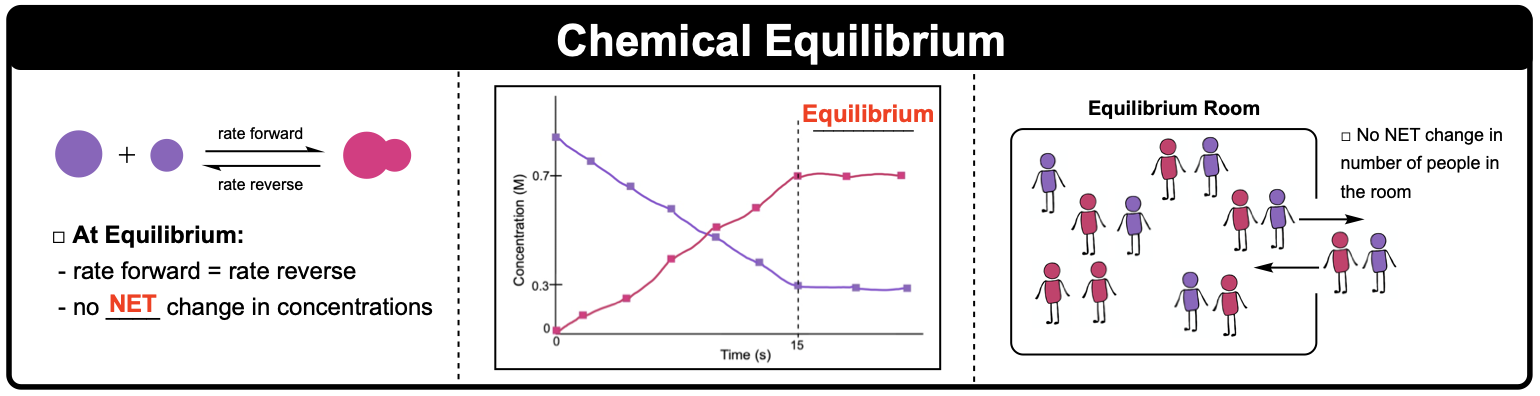Clutch Prep is now a part of Pearson
Ch.14 - Chemical EquilibriumWorksheetSee all chapters

# Intro to Chemical Equilibrium

See all sections
Sections
Intro to Chemical Equilibrium
Equilibrium Constant (K)
Equilibrium Constant Calculations
Kp and Kc
Using Hess's Law To Determine K
Calculating K For Overall Reaction
Le Chatelier's Principle
ICE Charts
Reaction Quotient

Most chemical reactions never go to completion, instead reach a chemical equilibrium.

###### Chemical Equilibrium

Concept #1: Intro to Chemical Equilibrium Concept 1

A reaction reaches equilibrium once the rate of the forward reaction equals the rate of the reverse reaction. Once at equilibrium there is no net change in the concentration of reactants or products.Example #1: Describe what happens when reaction reaches chemical equilibrium.

a) reaction stops

b) reactants form products as fast as products form reactants

c) the collision frequencies of products and reactants are identical

d) rates of forward and reverse reactions are equal to zero

Practice: Which of the following does not represent a heterogenous equilibrium?

I) CH4(g) + 2 O2(g) ⇌ CO2(g) + 2 H2O(g)
II) CO2(g) + C(s) ⇌ 2 CO(g)
III) 2 H2O(l) ⇌ 2 H2(g) + O2(g)
IV) CH3COOH(aq) + H2O(l) ⇌ CH3COO(aq) + H3O+(aq)